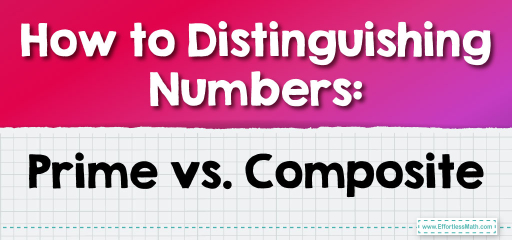# How to Distinguishing Numbers: Prime vs. Composite

Numbers are the building blocks of mathematics, and understanding their fundamental nature is crucial.Among the classifications of numbers, prime and composite numbers stand out due to their unique properties. In this guide, we’ll dive deep into the world of prime and composite numbers, exploring their definitions, characteristics, and how to identify them.

## Step-by-step Guide to Distinguishing Numbers; Prime vs. Composite:

1. Understanding Prime Numbers:

A prime number is a number greater than 1 that has no positive divisors other than 1 and itself. In other words, if a number is prime, it cannot be divided evenly by any other number except for 1 and itself.

2. Understanding Composite Numbers:

A composite number is a positive integer that has at least one positive divisor other than one or itself. Essentially, it’s any number greater than 1 that isn’t prime.

3. Identifying Prime Numbers:

To determine if a number is prime:

– Check if it’s less than 2. If so, it’s neither prime nor composite.

– For numbers greater than 2, try dividing it by all numbers up to the square root of that number. If none of them divide the number without leaving a remainder, it’s prime.

4. Identifying Composite Numbers:

If a number is not prime and is greater than 1, it’s composite.

### Example 1:

Is 7 a prime or composite number?

Solution:

7 is only divisible by 1 and 7. Therefore, 7 is a prime number.

The Absolute Best Book for 5th Grade Students

### Example 2:

Is 10 a prime or composite number?

Solution:

10 is divisible by 1, 2, 5, and 10. Since it has divisors other than 1 and itself, 10 is a composite number.

### Practice Questions:

1. Is 2 a prime or composite number?

2. Is 15 a prime or composite number?

3. Is 19 a prime or composite number?

A Perfect Book for Grade 5 Math Word Problems!

1. Prime

2. Composite

3. Prime

The Best Math Books for Elementary Students

### What people say about "How to Distinguishing Numbers: Prime vs. Composite - Effortless Math: We Help Students Learn to LOVE Mathematics"?

No one replied yet.

X
51% OFF

Limited time only!

Save Over 51%

SAVE $15 It was$29.99 now it is \$14.99